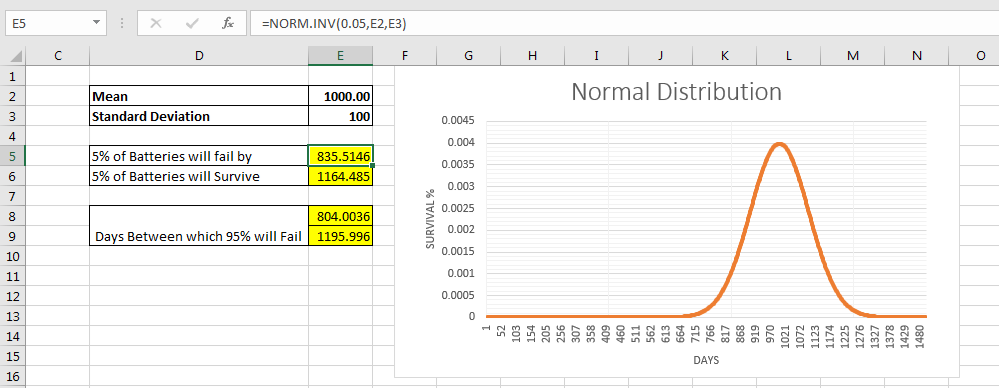# How to Use Excel NORM.INV Function

The NORM.INV function is used to get INVERSE CUMULATIVE DISTRIBUTION FUNCTION (ICDF). The ICDF is used to know the value associated with a probability, given the mean and standard deviation. We will understand in an example.Syntax of NORM.INV

 =NORM.INV(probability, mean, standard deviation)

Probability: the probability quotient. Mostly a fraction less then 1 and greater than 0.

Mean: the mean of data,

Standard deviation. The standard deviation of data.

Let’s see an example to make things clear

## Example: Set Warranty For An Electronic Product

Let’s say, you work in a mobile phone company. On an average, the battery fails after 1000 days with standard deviation of 100.

Find the the days by which 5% (0.05) of batteries will fail.
So we have
Probability:= 0.05
Mean:= 1000
Standard Deviation:= 100
Use NORM.INV function

 =NORM.INV(0.05,1000,100)

The above formula returns 835.5. It means 5% of batteries will expire within 836 days. It is the ICDF of 0.05 in above example. The manual calculation is really complex. Excel NORM.INV function makes it simple.

Find the the days by which 5% (0.05) of batteries will survive.

Now we need to calculate the number of days by which 5% batteries will survive. To do so we need to calculate ICDF of 95% of Failure. This will be the number of days by which 5% batteries will survive.
So we have
Probability:= 0.95
Mean:= 1000
Standard Deviation:= 100
Use NORM.INV function

 =NORM.INV(0.95,1000,100)

This returns 1164.5. This means 5% of batteries will survive after 1165 days.
Find the the days by which 95% (0.95) of batteries will fail.
Earlier we calculated, before and after days by which 5% of batteries will fail. Now we need to calculated days in which 95% of batteries will fail.
For that we need to leave 2.5% on each side of normal distribution. So we will calculate the ICDF of 2.5% and ICDF of 97.5% using Excel NORM.INV.
The number of days we will get from both ICDFs will be the day interval in which 95% of batteries will fail.
So we have here
Probability:= 0.025
Mean:= 1000
Standard Deviation:= 100
Use NORM.INV function

 =NORM.INV(0.025,1000,100)

This gives us 804.
Next we have
Probability:= 0.975
Mean:= 1000
Standard Deviation:= 100
Use NORM.INV function

 =NORM.INV(0.975,1000,100)

This gives us 1196.
So number of days between which 95% of batteries will fail is 804 to 1196.

Now we can use this for our warranty for batteries.

So yeah guys, this how you can use NORM.INV function in excel to save time and do crucial analysis easily. This function was introduced in excel 2010. The NORMINV function was available in earlier excel version. It is still available in excel 2016 and above but excel recommends to use NORM.INV function.

I am not an statistics expert and above example is just to explain the use of NORM.INV function. The statical meaning can be different from what I told. But the use is accurate. Let me know if you have any doubt regarding this function or any other function of excel. The comments section is all yours.

Terms and Conditions of use

The applications/code on this site are distributed as is and without warranties or liability. In no event shall the owner of the copyrights, or the authors of the applications/code be liable for any loss of profit, any problems or any damage resulting from the use or evaluation of the applications/code.